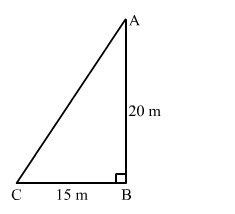# A ladder is placed in such a way that its foot is at a distance of 15 m from a wall and its top reaches a window 20 m above the ground.

Question:

A ladder is placed in such a way that its foot is at a distance of 15 m from a wall and its top reaches a window 20 m above the ground. Find the length of the ladder.

Solution:

Let the height of the window from the ground and the distance of the foot of the ladder from the wall be AB and BC, respectively.
We have:
AB = 20 m and BC = 15 m
Applying Pythagoras theorem in right-angled triangle ABC, we get:$A C^{2}=A B^{2}+B C^{2}$

$\Rightarrow A C=\sqrt{20^{2}+15^{2}}$

$=\sqrt{400+225}$

$=\sqrt{625}$

$=25 m$

Hence, the length of the ladder is 25 m.